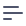# Types and Formats

## Measures Types

This section describes the various types that can be assigned to a measure. A measure can only have one type.

### number

The `sql` parameter is required and can take any valid SQL expression that results in a number or integer. Type `number` is usually used, when performing arithmetic operations on measures. Learn more about Calculated Measures.

``````purchasesRatio: {
sql: `\${purchases} / \${count} * 100.0`,
type: `number`,
format: `percent`
}
``````

You can put any sql into `number` measure as soon as it's an aggregate expression:

``````ratio: {
sql: `sum(\${CUBE}.amount) / count(*)`,
type: `number`
}
``````

### count

Performs a table count, similar to SQL’s `COUNT` function. However, unlike writing raw SQL, Cube.js will properly calculate counts even if your query’s joins will produce row multiplication. You do not need to include a `sql` parameter for this type.

`drillMembers` parameter is commonly used with type `count`. It allows users to click on the measure in the UI and inspect individual records that make up a count. Learn more about Drill Downs.

``````numberOfUsers: {
type: `count`,
// optional
drillMembers: [id, name, email, company]
}
``````

### countDistinct

Calculates the number of distinct values in a given field. It makes use of SQL’s `COUNT DISTINCT` function.

The `sql` parameter is required and can take any valid SQL expression that results in a table column, or interpolated Javascript expression.

``````uniqueUserCount: {
sql: `user_id`,
type: "countDistinct"
}
``````

### countDistinctApprox

Calculates approximate number of distinct values in a given field. Unlike `countDistinct` measure type, `countDistinctApprox` is decomposable aggregate function or Additive. This allows it's usage in Additive rollup pre-aggregations which are much more versatile than Not Additive ones. It uses special SQL backend dependent functions to estimate distinct counts. It usually based on HyperLogLog or similar algorithms. Where possible Cube.js will use multi-stage HLL which significantly improves calculation of distinct counts on scale.

The `sql` parameter is required and can take any valid SQL expression.

``````uniqueUserCount: {
sql: `user_id`,
type: "countDistinctApprox"
}
``````

### sum

Adds up the values in a given field. It is similar to SQL’s `SUM` function. However, unlike writing raw SQL, Cube.js will properly calculate sums even if your query’s joins will result in row duplication.

The `sql` parameter is required and can take any valid SQL expression that results in a numeric table column, or interpolated Javascript expression. `sql` parameter should contain only expression to sum without actual aggregate function.

``````revenue: {
sql: `\${chargesAmount}`,
type: `sum`
}
``````
``````revenue: {
sql: `amount`,
type: `sum`
}
``````
``````revenue: {
sql: `fee * 0.1`,
type: `sum`
}
``````

### avg

Averages the values in a given field. It is similar to SQL’s AVG function. However, unlike writing raw SQL, Cube.js will properly calculate averages even if your query’s joins will result in row duplication.

The sql parameter for type: average measures can take any valid SQL expression that results in a numeric table column, or interpolated Javascript expression.

``````averageTransaction: {
sql: `\${transactionAmount}`,
type: `avg`
}
``````

### min

Type of measure `min` is calculated as a minimum of values defined in `sql`.

``````dateFirstPurchase: {
sql: `date_purchase`,
type: `min`
}
``````

### max

Type of measure `max` is calculated as a maximum of values defined in `sql`.

``````dateLastPurchase: {
sql: `date_purchase`,
type: `max`
}
``````

### runningTotal

Type of measure `runningTotal` is calculated as summation of values defined in `sql`. Use it to calculate cumulative measures.

``````totalSubscriptions: {
sql: `subscription_amount`,
type: `runningTotal`
}
``````

## Measures Formats

When creating a measure you can explicitly define the format you’d like to see as output.

### percent

`percent` is used for formatting numbers with a percent symbol.

``````purchaseConversion: {
sql: `\${purchase}/\${checkout}*100.0`,
type: `number`,
format: `percent`
}
``````

### currency

`currency` is used for monetary values.

``````totalAmount: {
sql: `amount`,
type: `runningTotal`,
format: `currency`
}
``````

## Dimensions Types

This section describes the various types that can be assigned to a dimension. A dimension can only have one type.

### time

In order to be able to create time series charts, Cube.js needs to identify time dimension which is a timestamp column in your database.

You can define several time dimensions in schemas and apply each when creating charts. Note that type of target column should be TIMESTAMP. Please use this guide if your datetime information stored as a string.

``````completedAt: {
sql: `completed_at`,
type: `time`
}
``````

### string

`string` is typically used with fields that contain letters or special characters. The `sql` parameter is required and can take any valid SQL expression.

The following JS code creates a field `fullName` by combining 2 fields: `firstName` and `lastName`:

``````fullName: {
sql: `CONCAT(\${firstName}, ' ', \${lastName})`,
type: `string`
}
``````

### number

`number` is typically used with fields that contain number or integer.

``````amount: {
sql: `amount`,
type: `number`
}
``````

### boolean

`boolean` is used with fields that contain boolean data or data coercible to boolean. For example:

``````isEnabled: {
sql: `is_enabled`,
type: `boolean`
}
``````

### geo

`geo` dimension is used to display data on the map. Unlike other dimension types it requires to set two fields: latitude and longitude.

``````location: {
type: `geo`,
latitude: {
sql: `\${CUBE}.latitude`,
},
longitude: {
sql: `\${CUBE}.longitude`
}
}
``````

## Dimensions Formats

### imageUrl

`imageUrl` is used for displaying images in table visualization. In this case `sql` parameter should contain full path to the image.

``````image: {
sql: `CONCAT('https://img.example.com/id/', \${id})`,
type: `string`,
format: `imageUrl`
}
``````

### id

`id` is used for IDs. It allows to eliminate applying of comma for 5+ digit numbers which is default for type `number`. The `sql` parameter is required and can take any valid SQL expression.

``````image: {
sql: `id`,
type: `number`,
format: `id`
}
``````

### currency

`currency` is used for monetary values.

``````amount: {
sql: `abount`,
type: `number`,
format: `currency`
}
``````

### percent

`percent` is used for formatting numbers with a percent symbol.

``````openRate: {
sql: `COALESCE(100.0 * \${uniqOpenCount} / NULLIF(\${deliveredCount}, 0), 0)`,
type: `number`,
format: `percent`
}
``````